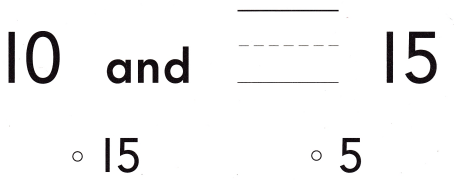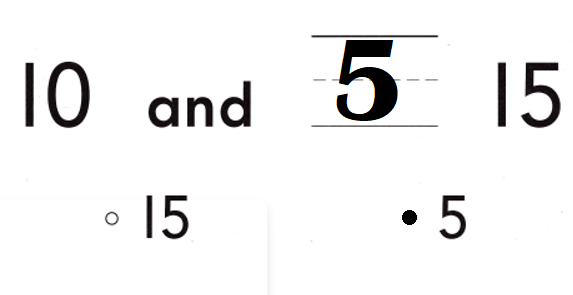# Texas Go Math Kindergarten Lesson 8.2 Answer Key Count and Write 16 and 17

Refer to our Texas Go Math Kindergarten Answer Key Pdf to score good marks in the exams. Test yourself by practicing the problems from Texas Go Math Kindergarten Lesson 8.2 Answer Key Count and Write 16 and 17.

## Texas Go Math Kindergarten Lesson 8.2 Answer Key Count and Write 16 and 17

Explore

DIRECTIONS: Count and tell how many. Trace and write the numbers and the words.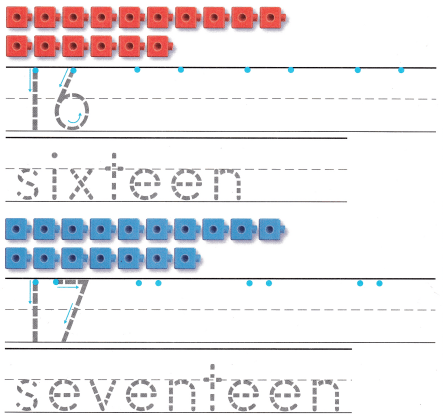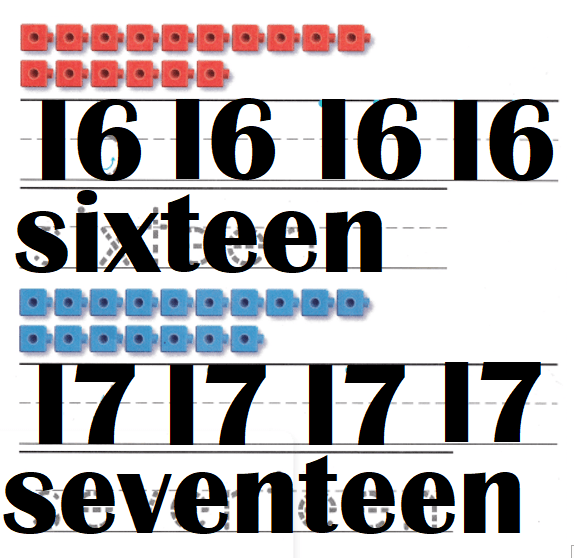Explanation:
Number of red cubes = 16 or Sixteen.
Number of blue cubes = 17 or Seventeen.

Share and Show

DIRECTIONS: 1. Count and tell how many. Practice writing the numbers. 2. Count and tell how many. Write the number. 3. Look at the ten frames in Exercise 2. Write how many red squares. Write how many blue squares. Write how many in all.

Question 1.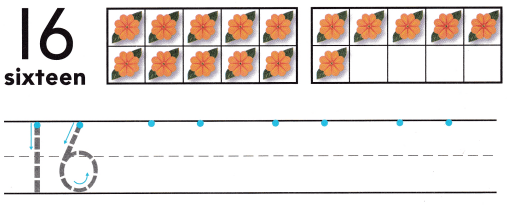Explanation:
Number of flowers = 16 or Sixteen.

Question 2.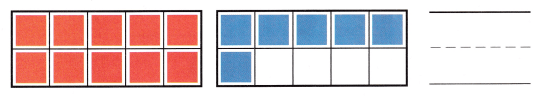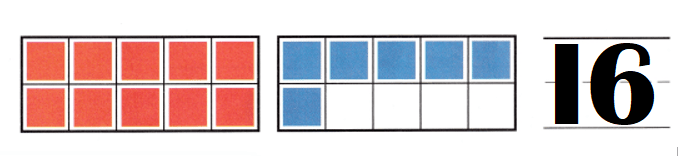Explanation:
Number of red cubes = 10.
Number of blue cubes = 6.
Total number of cubes = Number of red cubes + Number of blue cubes
= 10 + 6
= 16 or Sixteen.

Question 3.Explanation:
Number of red cubes = 10.
Number of blue cubes = 6.
Total number of cubes = Number of red cubes + Number of blue cubes
= 10 + 6
= 16 or Sixteen.

DIRECTIONS: 4. Count and tell how many. Practice writing the numbers. 5. Count and tell how many. Write the number. 6. Look at the ten frames in Exercise 5. Write how many red squares. Write how many blue squares. Write how many in all.

Question 4.Explanation:
Number of flowers = 10 + 7 = 17 or Seventeen.

Question 5.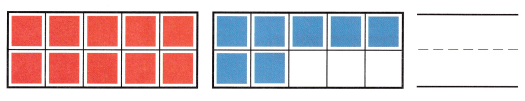Explanation:
Number of red cubes = 10.
Number of blue cubes = 7.
Total number of cubes = Number of red cubes + Number of blue cubes
= 10 + 7
= 17 or Seventeen.

Question 6.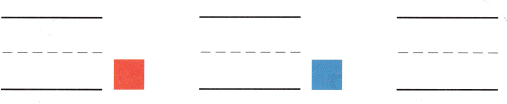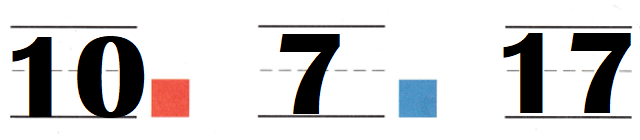Explanation:
Number of red cubes = 10.
Number of blue cubes = 7.
Total number of cubes = Number of red cubes + Number of blue cubes
= 10 + 7
= 17 or Seventeen.

HOME ACTIVITY • Ask your child to count and write the number for a set of 16 or 17 objects, such as macaroni pieces or buttons.Explanation:
My kid used buttons and counted them..
Number of red buttons = 16 or Sixteen.
Number of yellow buttons = 17 or Seventeen.

DIRECTIONS: 7. Circle a number. Draw more flowers to show that number. 8. Draw a set of 16 objects. Circle 10 of the objects. How many more objects are there? Write the number. 9. Choose the correct answer. What number goes in the blank to make 17?

Problem Solving
Question 7.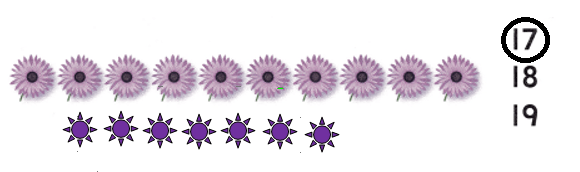Explanation:
Number of flowers = 10 + 7 = Seventeen.

Question 8.Explanation:
Number of apples = 10 + 6 = 16 or Sixteen.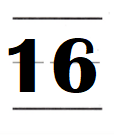Question 9.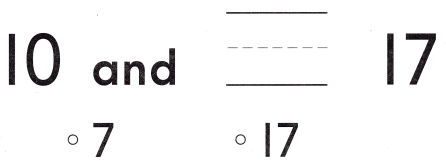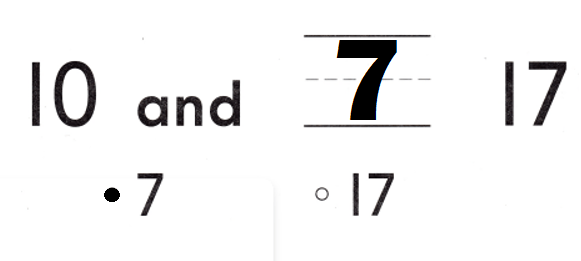Explanation:
10 + 7 = 17 or Seventeen.

### Texas Go Math Kindergarten Lesson 8.2 Homework and Practice Answer Key

DIRECTIONS: 1-2. Count and tell how many. Write the numbers.

Question 1.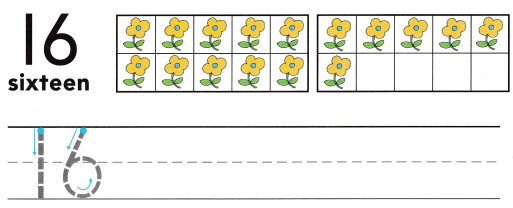Explanation:
Number of flowers = 16 or Sixteen.

Question 2.Explanation:
Number of Triangles = 17 or Seventeen.

DIRECTIONS: 3-5. Choose the correct answer. What number goes in the blank?

Lesson Check
Question 3.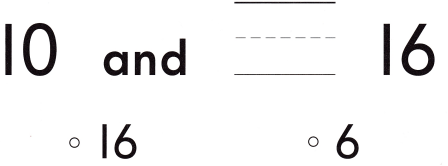Explanation:
10 + 6 = 16.

Question 4.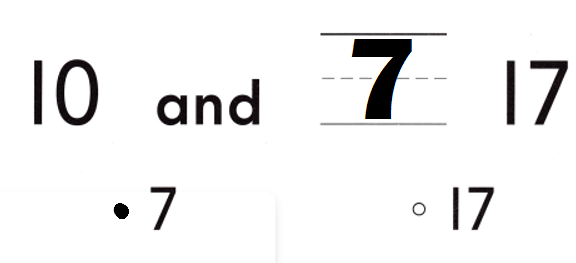Explanation:
10 + 7 = 17 or Seventeen.

Question 5.